# ML using scikit learn¶

## Predicting absenteeism¶

A large problem within organisations is how to motivate their employees. This is a continuation of the previous blog where we went through various feature engineering methods to come up with a comprehensive dataset. In this blog, we will use this data in order to predict employment absenteeism. The goal is to identify who are likely to be absent in the near future. This blog doesn't go through the machine learning concepts, or business logic, but implementation of machine learning using scikit-learn package in python.

As a first step, let us load and look at the data.

``````import warnings
warnings.filterwarnings('ignore', category=FutureWarning)

import matplotlib.pyplot as plt
import seaborn as sns
import plotly.express as px
import pandas as pd
import numpy as np
%matplotlib inline
``````
``````pd.set_option('display.max_columns', None)

df['date'] = pd.to_datetime(df.date)
``````
employee date last_likes last_dislikes feedbackType likes_till_date dislikes_till_date last_2_likes last_2_dislikes days_since_last_comment last_vote timezone stillExists no_of_days_since_first_vote no_of_votes_till_date perc_days_voted avg_vote_till_date avg_vote last_2_votes_avg days_since_last_vote employee_joined_after_jun17 countdown_to_last_day reason on_leave no_leaves_till_date last_2_days_leaves previous_day_leave weekday month day week
0 17r 2018-05-29 0 0 0 0 0 0 0 0 3 Europe/Madrid 1 1 1 1.0 3.0 2.121212 3.0 0 1 999 NaN 0 0 0 0 Tuesday May 29 1
1 17r 2018-05-30 0 0 0 0 0 0 0 0 3 Europe/Madrid 1 1 1 1.0 3.0 2.121212 3.0 0 1 999 NaN 0 0 0 0 Wednesday May 30 2
2 17r 2018-05-31 0 0 0 0 0 0 0 0 3 Europe/Madrid 1 2 1 1.0 3.0 2.121212 3.0 1 1 999 NaN 0 0 0 0 Thursday May 31 3
3 17r 2018-01-06 0 0 0 0 0 0 0 0 3 Europe/Madrid 1 3 2 0.5 3.0 2.121212 3.0 0 1 999 NaN 0 0 0 0 Friday Jun 1 1
4 17r 2018-02-06 0 0 0 0 0 0 0 0 3 Europe/Madrid 1 4 2 0.5 3.0 2.121212 3.0 0 1 999 NaN 0 0 0 0 Saturday Jun 2 2

## Base model¶

This data is at an employee-day level, and we can predict if any employee will take leave on any particular date. As very few employees take leave on any particular day, we will have a highly imbalanced dataset.

``````1-df.on_leave.mean()
``````
``````0.9514513766404592
``````

This indicates that only 5% of the dataset contains information about employees taking leaves. This means that if we predicted that all the employees are not taking a leave (class 0), we would be 95% accurate, but such prediction is not useful. This is the base model, and we need to see to it that we have accuracy greater than 95%.

## Decision Tree Classifier¶

``````from sklearn.tree import DecisionTreeClassifier, plot_tree
from sklearn.model_selection import train_test_split, GridSearchCV
from sklearn.metrics import classification_report, plot_confusion_matrix, confusion_matrix, accuracy_score, roc_curve, roc_auc_score
``````

From the different features that we have created, we are selecting what we think will be relevant features for predicting which employee might be absent.

``````indep_vars = ['last_likes', 'last_dislikes', 'feedbackType',
'likes_till_date', 'dislikes_till_date', 'last_2_likes', 'last_2_dislikes',
'days_since_last_comment', 'last_vote', 'timezone', 'stillExists',
'perc_days_voted', 'avg_vote_till_date', 'avg_vote', 'last_2_votes_avg',
'days_since_last_vote', 'employee_joined_after_jun17', 'countdown_to_last_day',
'no_leaves_till_date', 'weekday', 'month']
``````

The dependent variable is on_leave. We are also creating dummy variables that represent categorical data.

``````data_targets = df['on_leave'].astype('int')
data_features = pd.get_dummies(df[indep_vars], prefix = "_", drop_first= True)
``````

To prevent overfitting, we are splitting the data into test data and train data. Test data has 30% of the data (selected randomly) while the train data has the remaining 70% on which we train the model. This model is tested against the test data to validate for overfitting.

``````x_train, x_test, y_train, y_test = train_test_split(data_features, data_targets, test_size=.30, random_state=35, \
stratify=data_targets)
``````

Before building an ensemble of models, we can build a decision tree model and understand if the features we have selected perform the classification reasonably.

``````tree_clf = DecisionTreeClassifier(random_state=35, max_depth=3, class_weight="balanced").fit(x_train,y_train)
``````
``````def plot_feature_importance(model):
fs, ax = plt.subplots(1, figsize=(20,10))
number_of_features = x_train.shape
plt.barh(range(number_of_features), model.feature_importances_, align='center')
plt.yticks(np.arange(number_of_features),x_train.columns)
plt.xlabel("Feature Importance")
plt.ylabel("Feature")
plot_feature_importance(tree_clf)
``````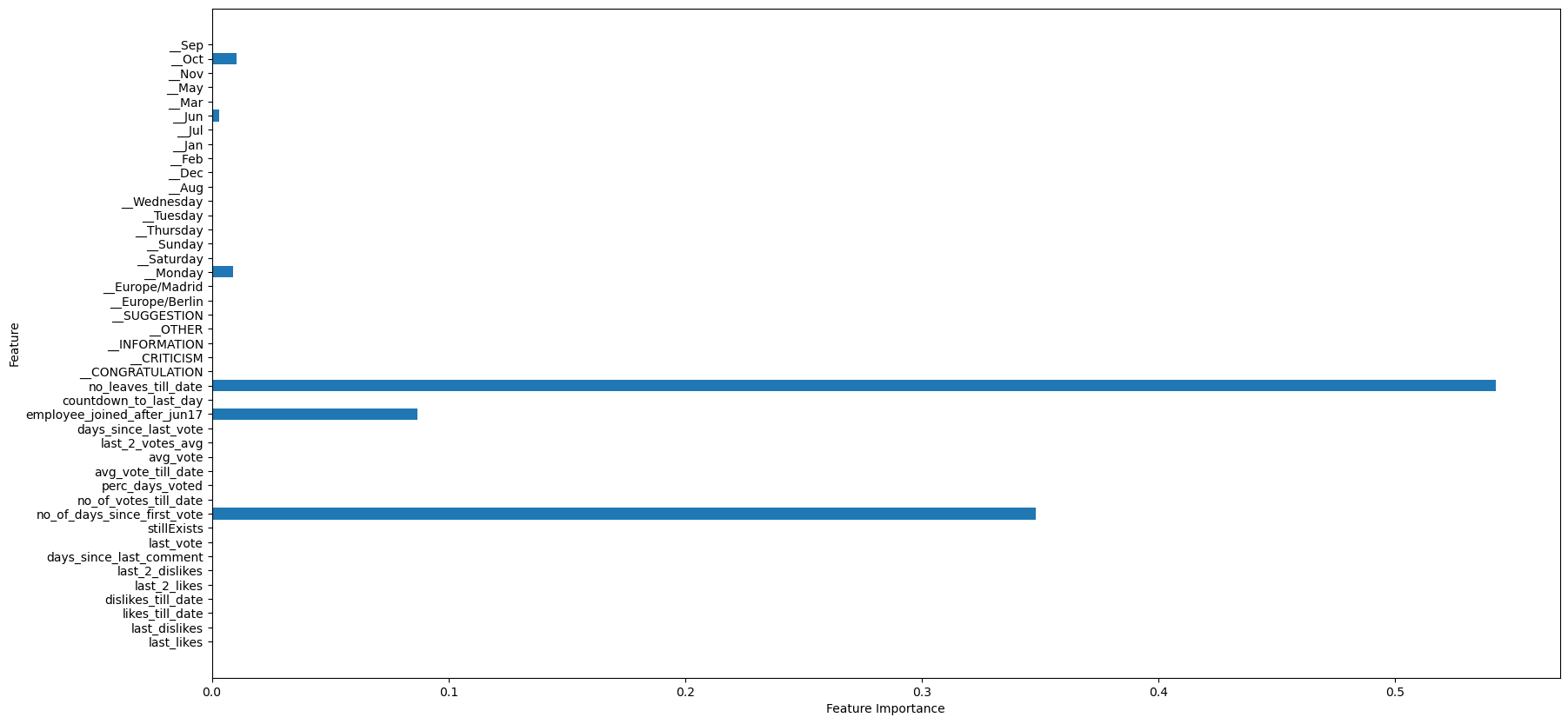``````plt.figure(figsize=(20,20))
plot_tree(tree_clf,  fontsize=10, feature_names = x_train.columns)
plt.show()
``````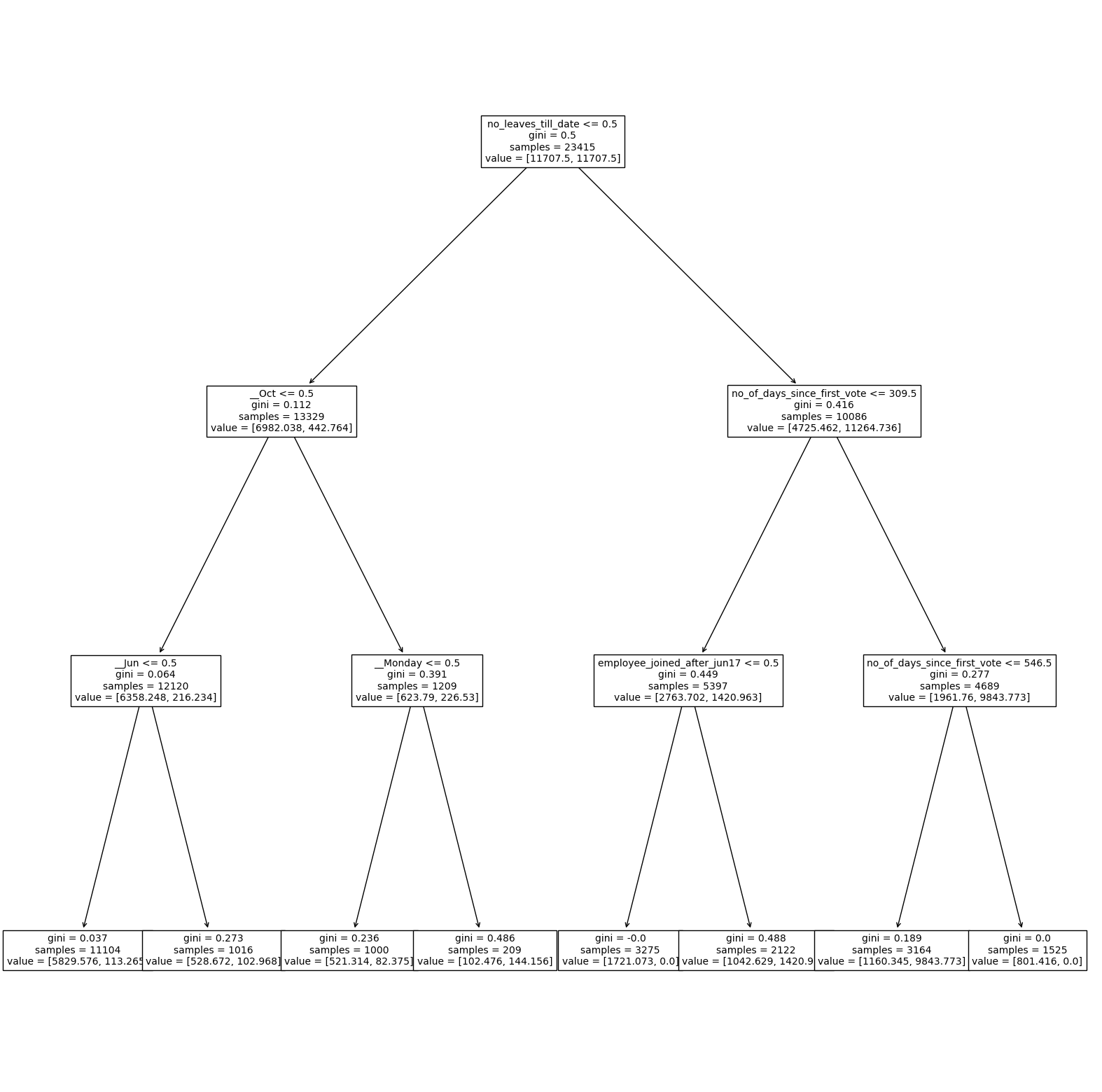We can observe that the features that are important make reasonable sense. 1. Number of leaves till date: The number of leaves that an employee has taken already will affect the future leaves that a person would take 2. number of days since first vote (proxy to the employee tenure), employee_joined_after_Jun17 (proxy to newer employees) are significant, and we have seen these trends in the visualizations in the feature engineering section 3. As seen in the visualisations, employees took leaves during months like June and October which have come up as significant in the analysis

We can see the model is not overfit with similar accuracy across test and train datasets. The confusion matrix is plotted below:

``````plt.rcParams["figure.figsize"] = (5, 5)
plot_confusion_matrix(tree_clf, x_test, y_test)
print('Accuracy on train data is', round(tree_clf.score(x_train, y_train),2))
print('Accuracy on test data is', round(tree_clf.score(x_test, y_test),2))
``````
``````Accuracy on train data is 0.81
Accuracy on test data is 0.81
``````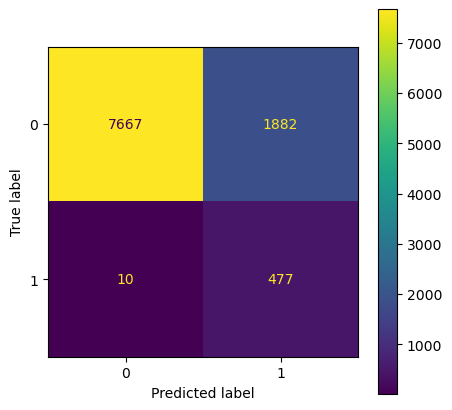``````y_test_pred = tree_clf.predict(x_test)
print(classification_report(y_test, y_test_pred))
``````
``````              precision    recall  f1-score   support

0       1.00      0.80      0.89      9549
1       0.20      0.98      0.34       487

accuracy                           0.81     10036
macro avg       0.60      0.89      0.61     10036
weighted avg       0.96      0.81      0.86     10036
``````

We can observe that the precision is just 20% (on classifying when an employee will take a leave). This is due to imbalance of classes. This can be rectified by many ways, one of which includes under-sampling. For other methods, refer the blog on handling imbalanced classes. We are also using GridSearch to find the best tuning parameters and n-fold cross validation.

### Downsampling¶

``````from imblearn.pipeline import Pipeline, make_pipeline
from imblearn.under_sampling import RandomUnderSampler
param_grid_dt = {
'classification__criterion': ['gini', 'entropy'],
'classification__max_depth': [2,5,10],
'classification__max_features':[0.2, 0.5, 0.8]
}
model_dt_p = Pipeline([
('sampling', RandomUnderSampler()), # While training, downsample the majority class randomly
('classification', DecisionTreeClassifier()) # Train and predict using rf classification model
])
model_dt = GridSearchCV(model_dt_p, param_grid_dt, cv=5)
model_dt.fit(x_train, y_train)
``````
``````GridSearchCV(cv=5,
estimator=Pipeline(steps=[('sampling', RandomUnderSampler()),
('classification',
DecisionTreeClassifier())]),
param_grid={'classification__criterion': ['gini', 'entropy'],
'classification__max_depth': [2, 5, 10],
'classification__max_features': [0.2, 0.5, 0.8]})
``````
``````y_test_pred = model_dt.predict(x_test)
print(classification_report(y_test, y_test_pred))
plot_confusion_matrix(model_dt, x_test, y_test)
print('Accuracy on train data is', round(model_dt.score(x_train, y_train),2))
print('Accuracy on test data is', round(model_dt.score(x_test, y_test),2))
``````
``````              precision    recall  f1-score   support

0       1.00      0.96      0.98      9549
1       0.54      0.98      0.69       487

accuracy                           0.96     10036
macro avg       0.77      0.97      0.84     10036
weighted avg       0.98      0.96      0.96     10036

Accuracy on train data is 0.96
Accuracy on test data is 0.96
``````Here we observe that with a simple decision tree classifier, we already reach an accuracy above 95% (base accuracy) with good precision and recall values. This model is better than our base model. We could further improve it by using ensemble methods which we will look into later in the blog.

``````def print_stats(model):
print('Best params for the model', model.best_params_)
print('Best score on the cross validation data', round(model.best_score_, 2))
print('Accuracy on train data', round(model.score(x_train, y_train),2))
print('Accuracy on test data', round(model.score(x_test, y_test),2))
print_stats(model_dt)
plt.show()
``````
``````Best params for the model {'classification__criterion': 'entropy', 'classification__max_depth': 10, 'classification__max_features': 0.5}
Best score on the cross validation data 0.96
Accuracy on train data 0.96
Accuracy on test data 0.96
``````
``````y_test_pred = model_dt.predict(x_test)
print(classification_report(y_test, y_test_pred))
``````
``````              precision    recall  f1-score   support

0       1.00      0.96      0.98      9549
1       0.54      0.98      0.69       487

accuracy                           0.96     10036
macro avg       0.77      0.97      0.84     10036
weighted avg       0.98      0.96      0.96     10036
``````

## Multiple models¶

After manually applying a few models, we can scale up to use grid search method to search for the best classifier and parameters in a quick and efficient manner. We can use Logistic Regression, Multinomial Naive Bayes, KNN Classifiers, Ensemble models and any model in the sklearn library.

``````from sklearn.linear_model import LogisticRegression
from sklearn.naive_bayes import MultinomialNB
from sklearn.neighbors import KNeighborsClassifier
from sklearn.ensemble import RandomForestClassifier,GradientBoostingClassifier
from sklearn.pipeline import Pipeline
``````
``````# setting up models

clf1 = LogisticRegression(random_state=35)
clf2 = MultinomialNB()
clf3 = KNeighborsClassifier()
clf4 = DecisionTreeClassifier(random_state=35)
clf5 = RandomForestClassifier(random_state = 35)
clf6 = GradientBoostingClassifier(random_state = 35)
``````
``````# setting up hyperparameters

# Hyperparameters for Logistic regression https://scikit-learn.org/stable/modules/linear_model.html#logistic-regression
hyperparam1 = {}
hyperparam1['classifier__C'] = [10**-4, 10**-1, 10**0] # The hyper-parameter is C and its inside 'classifier' part of pipeline
hyperparam1['classifier__penalty'] = ['l1', 'l2'] # The hyper-parameter is penalty and its inside 'classifier' part of pipeline
hyperparam1['classifier__class_weight'] = [None, 'balanced']
hyperparam1['classifier'] = [clf1]

# Hyperparameters for Naive Bayes
hyperparam2 = {}
hyperparam2['classifier__alpha'] = [10**0,10**4]
hyperparam2['classifier'] = [clf2]

# Hyperparameters for KNN
hyperparam3 = {}
hyperparam3['classifier__n_neighbors'] = [2, 5, 10]
hyperparam3['classifier'] = [clf3]

# Hyperparameters for Decision Trees
hyperparam4 = {}
hyperparam4['classifier__max_depth'] = [2,5,10, None]
hyperparam4['classifier__min_samples_split'] = [2, 5, 10]
hyperparam4['classifier__class_weight'] = [None, 'balanced']
hyperparam4['classifier'] = [clf4]

# Hyperparameters for Random Forest
hyperparam5 = {}
hyperparam5['classifier__n_estimators'] = [100, 250]
hyperparam5['classifier__max_depth'] = [2,5,10]
hyperparam5['classifier__class_weight'] = [None, 'balanced']
hyperparam5['classifier'] = [clf5]

# Hyperparameters for Gradient Boosting
hyperparam6 = {}
hyperparam6['classifier__n_estimators'] = [100, 250]
hyperparam6['classifier__max_depth'] = [2, 5, 10]
hyperparam6['classifier__min_samples_split'] = [2, 5, 10]
hyperparam6['classifier'] = [clf6]

pipe = Pipeline([('classifier',clf1)])
hyperparam_total = [hyperparam1, hyperparam2, hyperparam3, hyperparam4, hyperparam5, hyperparam6]
``````
``````%%time
# Record how long the search takes

# Train the grid search model
model_grid_search = GridSearchCV(pipe, hyperparam_total, cv=3, n_jobs=-1, scoring='roc_auc').fit(x_train, y_train)
``````
``````C:\ProgramData\Anaconda3\lib\site-packages\sklearn\model_selection\_search.py:918: UserWarning: One or more of the test scores are non-finite: [       nan 0.76530486        nan 0.78573417        nan 0.76227159
nan 0.81272732        nan 0.7669747         nan 0.81235721
nan        nan 0.9792672  0.98689225 0.98684304 0.80196935
0.80196935 0.80196935 0.9807685  0.9807685  0.98227199 0.974107
0.97684471 0.98018112 0.96721555 0.9697727  0.97256904 0.87247414
0.87247414 0.87247414 0.95249357 0.95249357 0.95249357 0.96331177
0.96410843 0.96467699 0.96485967 0.96883658 0.97032722 0.96982298
0.96802826 0.99322296 0.99325364 0.99761825 0.99778465 0.96814859
0.97048243 0.98832118 0.98900645 0.9941528  0.99483174 0.99417744
0.99651075 0.99417744 0.99651075 0.99417744 0.99651075 0.99771714
0.99782095 0.99764383 0.99792168 0.99770636 0.99797557 0.99532432
0.99816234 0.99575252 0.99821671 0.99738392 0.99794075]
warnings.warn(

Wall time: 22min 59s
``````
``````# Output the best estimator which is a random forecast classifier
model_grid_search.best_estimator_
``````
``````Pipeline(steps=[('classifier',
n_estimators=250,
random_state=35))])
``````

After running grid search to find the best parameters and models, we find the best model to be Gradient Boosting with the best parameters below.

``````# Output the parameters in the best estimator
model_grid_search.best_params_
``````
``````{'classifier': GradientBoostingClassifier(max_depth=10, min_samples_split=5, n_estimators=250,
random_state=35),
'classifier__max_depth': 10,
'classifier__min_samples_split': 5,
'classifier__n_estimators': 250}
``````

The accuracy on the cross validated data close to 99%.

``````round(model_grid_search.best_score_, 4)
``````
``````0.9982
``````

On the test data also, we have a near perfect precision, recall and accuracy.

``````y_test_pred = model_grid_search.predict(x_test)
print(classification_report(y_test, y_test_pred))
``````
``````              precision    recall  f1-score   support

0       1.00      1.00      1.00      9549
1       0.99      0.96      0.97       487

accuracy                           1.00     10036
macro avg       0.99      0.98      0.99     10036
weighted avg       1.00      1.00      1.00     10036
``````
``````plot_confusion_matrix(model_grid_search, x_test, y_test)
plt.show()
``````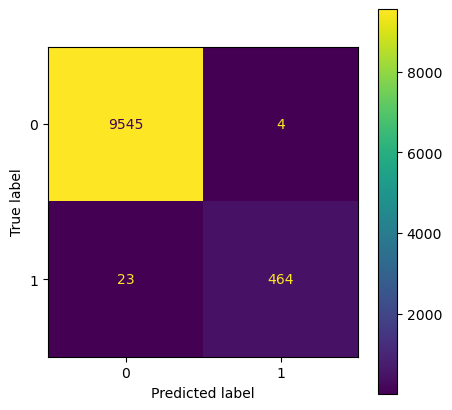The features that are used and their importances in the model is:

``````def print_most_imp_features(model, data_features):
feat_imp_df = pd.DataFrame({'columns' : data_features.columns, 'importance':(model.best_estimator_.named_steps["classifier"].feature_importances_*100).astype(int)})
return feat_imp_df[feat_imp_df.importance>0].sort_values('importance', ascending=False)
print_most_imp_features(model_grid_search, data_features)
``````
columns importance
18 no_leaves_till_date 28
9 no_of_days_since_first_vote 25
32 __Aug 8
41 __Oct 7
37 __Jun 6
12 avg_vote_till_date 5
11 perc_days_voted 3
13 avg_vote 3
5 last_2_dislikes 2
3 dislikes_till_date 1
6 days_since_last_comment 1
``````def plot_roc_curve(fpr, tpr, auc):
fig, ax = plt.subplots()
ax.plot(fpr, tpr)
ax.set(xlabel='False Positive Rate', ylabel='True Positive Rate')
ax.grid()
ax.text(0.6, 0.3, 'ROC AUC Score: {:.3f}'.format(auc),
lims = [np.min([ax.get_xlim(), ax.get_ylim()]), np.max([ax.get_xlim(), ax.get_ylim()])]
ax.plot(lims, lims, 'k--')
ax.set_xlim(lims)
ax.set_ylim(lims)
plt.title('ROC curve')
``````
``````auc = roc_auc_score(y_test, y_test_pred)
fpr, tpr, _ = roc_curve(y_test, y_test_pred)
plot_roc_curve(fpr, tpr, auc)
``````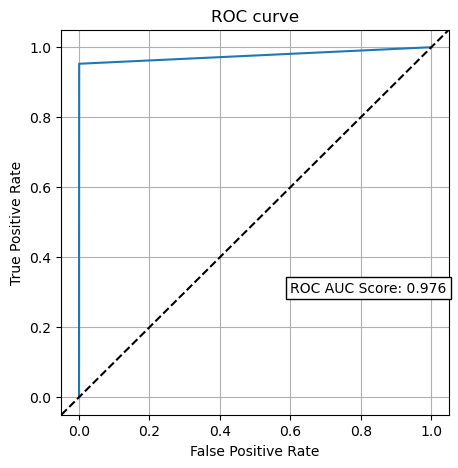## Predictions¶

Using this model, we can predict who is going to be absent in the next few days.

``````next_day_df = df[df.date == max(df.date)].reset_index()
next_day_df.no_of_days_since_first_vote += 1
next_day_df.days_since_last_vote += 1

day_name= ['Monday', 'Tuesday', 'Wednesday', 'Thursday', 'Friday', 'Saturday','Sunday']
month_name = [None, 'Jan', 'Feb', 'Mar', 'Apr', 'May', 'Jun', 'Jul', 'Aug', 'Sep', 'Oct', 'Nov', 'Dec']
next_day_df.date = next_day_df.date + pd.DateOffset(1)
next_day_df['weekday'] = next_day_df.date.dt.weekday.apply(lambda x:day_name[x])
next_day_df['month'] = next_day_df.date.dt.month.apply(lambda x:month_name[x])
next_day_df['week'] = next_day_df.date.dt.day

# Handle catogorical variables
next_day_df_predict = pd.get_dummies(next_day_df[indep_vars], prefix = "_")
next_day_df_predict = next_day_df_predict.reindex(columns=x_train.columns, fill_value=0)

prob_current = model_grid_search.predict_proba(next_day_df_predict)
next_day_df['leave_prob'] = prob_current[:,1]
``````

The top 5 employees who have the highest probability to be absent in the next day are:

``````next_day_df.sort_values('leave_prob', ascending = False).head(5)
``````
index employee date last_likes last_dislikes feedbackType likes_till_date dislikes_till_date last_2_likes last_2_dislikes days_since_last_comment last_vote timezone stillExists no_of_days_since_first_vote no_of_votes_till_date perc_days_voted avg_vote_till_date avg_vote last_2_votes_avg days_since_last_vote employee_joined_after_jun17 countdown_to_last_day reason on_leave no_leaves_till_date last_2_days_leaves previous_day_leave weekday month day week leave_prob
22 13699 DNY 2019-12-03 0 0 0 0 0 0 0 0 3 Europe/Madrid 1 651 229 0.352851 2.934498 2.936441 3.0 1 0 999 NaN 0 2 0 0 Tuesday Dec 12 3 9.382249e-10
34 20478 YDm 2019-12-03 19 0 CONGRATULATION 523 250 34 9 42 3 Europe/Madrid 1 651 537 0.827427 2.942272 2.943636 3.0 1 0 999 NaN 0 1 0 0 Tuesday Dec 12 3 7.326212e-10
38 22997 aQJ 2019-12-03 8 8 INFORMATION 237 71 56 10 163 3 Europe/Madrid 1 462 139 0.446945 3.071942 3.071942 3.0 151 1 999 NaN 0 14 0 0 Tuesday Dec 12 3 5.486968e-10
37 22508 aKP 2019-12-03 10 23 SUGGESTION 482 148 51 43 58 4 Europe/Madrid 1 651 103 0.173693 3.805825 3.805825 4.0 57 0 999 NaN 0 0 0 0 Tuesday Dec 12 3 4.874565e-10
12 7723 5WZ 2019-12-03 0 0 OTHER 38 7 0 0 7 3 Europe/Berlin 0 646 548 0.849612 2.958029 2.959436 3.0 1 0 999 NaN 0 31 0 0 Tuesday Dec 12 3 4.845051e-10

## Save Models¶

The last step is to save models for future deployment or run. Scikit-learn models are usually saved as pickle files.

``````import pickle
# Dump the trained model with Pickle
model_pkl_filename = 'classifier_employee_absenteeism.pkl'
# Open the file to save as pkl file
model_pkl = open(model_pkl_filename, 'wb')
pickle.dump(model_grid_search, model_pkl)
# Close the pickle instances
model_pkl.close()
``````
1. Scikit-learn documentation: link
2. Satyam Kumar, How to tune multiple ML models with GridSearchCV at once?: link
3. Notes and lectures, Machine Learning module, MSc Business analytics, Imperial College London, Class 2020-22
4. Harsha A, Shaked A, Artem G, Tebogo M, Gokhan M: The workforce of the future Workforce Analytics
5. Harsha A, Shaked A, Artem G, Tebogo M, Gokhan M: Predicting absenteeism Workforce Analytics